# Weierstrass formula

(diff) ← Older revision | Latest revision (diff) | Newer revision → (diff)

for the increment of a functional

A formula in the classical calculus of variations (cf. Variational calculus), defining the values of the functional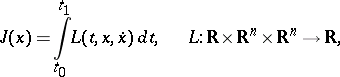in the form of a curvilinear integral of the Weierstrass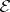-function. Let the vector functionbe an extremal of the functional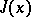, and let it be included in an extremal field with vector-valued field slope functionand action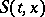, corresponding to this field (cf. Hilbert invariant integral). Weierstrass' formula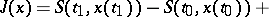(1)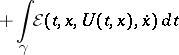applies to any curve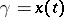in the domain covered by the field. In particular, if the boundary conditions of the curvesandare identical, i.e. if,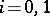, one obtains Weierstrass' formula for the increment of a functional: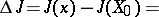(2)Formulas (1) and (2) are sometimes referred to as Weierstrass' fundamental theorem.

How to Cite This Entry:
Weierstrass formula. Encyclopedia of Mathematics. URL: http://encyclopediaofmath.org/index.php?title=Weierstrass_formula&oldid=16280
This article was adapted from an original article by V.M. Tikhomirov (originator), which appeared in Encyclopedia of Mathematics - ISBN 1402006098. See original article